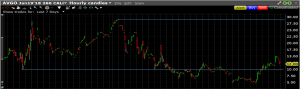25
Feb 18

## Volatility - king among option price determinants

Here we apply the simple probabilistic model developed previously to see how a call price depends on volatility. Assumption 1 and the method to find ranges of initial stock prices that can lead to ITM areas give Figure 1.Figure 1. Dependence on volatility

As before, we are interested in the event$\{K that the call with the strike price$K$ is in the money at expiration. In Figure 1,$S_1$ corresponds to volatility$\sigma_1$ and$S_2$ corresponds to volatility$\sigma_2$, where$\sigma_1<\sigma_2$$S_1$ is the highest stock price such that its area of influence (the base of the corresponding rectangle) does not contribute to the ITM area. Hence, only stock prices higher than$S_1$ do (these prices are indicated by the green arrow). The same goes for$S_2$, the range of prices that contribute to the ITM area being shown by the blue arrow. We see that the area corresponding to the larger volatility is wider, and therefore the corresponding call is more expensive.Figure 2. Call as a function of volatility

In Figure 1, the independent variable (on the horizontal axis) is time. Figure 2 from Mathematica illustrates the same dependence with volatility as the independent variable.

## The Black-Scholes formula doesn't tell the full story

In the Black-Scholes formula, the volatility parameter is fixed. In practice, it changes all the time. The dependence on volatility, when the latter is very large, can dominate all other determinants. This is best illustrated with an example.Figure 3. AVGO stock

Figure 3 is the chart of AVGO (symbol of Broadcom Ltd) in the period of high volatility 10/30/2017-1/8/2018. In general, it's difficult to pick exactly tops and bottoms. But suppose you were able to do so buying the stock at $248 and selling at$286. Usually stocks are traded in batches of 100. In that case, your profit would be (286-248)*100=3800 and the return would be 3800/24800=15.3%. Let's compare this return with the one you could have trading calls.Figure 4. AVGO 260 call

In Figure 4 you can see the behavior of 260 call option that expired on January 19, 2018. The period is the same and both charts are hourly charts. One call option commands 100 shares of stock. Again, assuming that you were able to pick the bottom and top exactly, your profit would be (29-10)*100=1900 and the return would be 1900/1000=190%. You would have a much higher return with a much smaller investment!

Note also that in the subsequent period, when the volatility and stock price both fell, the stock price did not fall below the previous low of $248, while the option price broke the previous low of$10 and hit \$5 at some point.

Conclusion. The key to trading options is to catch a moment when volatility is low and is about to jump or when it is high and is going to fall.